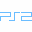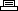Site SearchRed Faction 2Printer Friendly Version

```Master cheat code:
Enter Square, Circle, Triangle, Circle, Square, X, Triangle,
X at the cheats screen under the options menu.
All cheats will be unlocked.

Master option code:
Enter Triangle, Triangle, X, X, Square, Circle, Square,
Circle at the cheats screen under the options menu.
All normal game options will be unlocked.

Level select:
Enter Circle, Square, X, Triangle, Square, Circle, X, X at
the cheats screen under the options menu. Start a new game
and all levels will be unlocked.

Super health:
Enter X, X, Square, Triangle, Square, Triangle, Circle at
the cheats screen under the options menu.

Unlimited ammunition:
Enter Square, Triangle, X, Circle, Square, Circle, X,
Triangle at the cheats screen under the options menu.

Enter Circle, X, Circle, Square, X, Circle, X, Circle at
the cheats screen under the options menu.

Rapid rails:
Enter Circle, Square, Circle, Square, X, X, Triangle,
Triangle at the cheats screen under the options menu.

Enter Circle, Circle, Circle, Circle, Circle, Circle,
Circle, Circle at the cheats screen under the options menu.

Gibby explosions:
Enter Triangle, Circle, X, Square, Triangle, Circle, X,
Square at the cheats screen under the options menu.

Gibby ammunition:
Enter X, X, X, X, Square, Circle, X, X at the cheats

Fat mode:
Enter Circle, Circle, Circle, Circle, Triangle, X, Circle,
Circle at the cheats screen under the options menu.

Directors cut:
Enter Square, X, Circle, Triangle, Circle, X, Square,
Triangle at the cheats screen under the options menu.

Enter X, X, X, X, X, X, X, X at the cheats screen under

Wacky deaths:
Enter Triangle, Triangle, Triangle, Triangle, Triangle,
Triangle, Triangle, Triangle at the cheats screen under

Rain of fire:
Enter Square, Square, Square, Square, Square, Square,
Square, Square at the cheats screen under the options menu.

Explosive personality:
Enter X at the cheats screen under the options menu.
Press Use on someone and they will gib.

Joke message:
Enter Square, X, Square, X at the cheats screen under